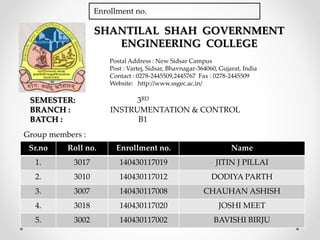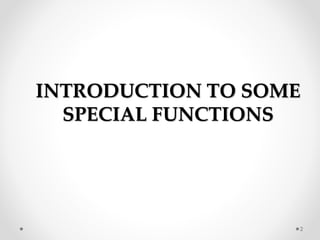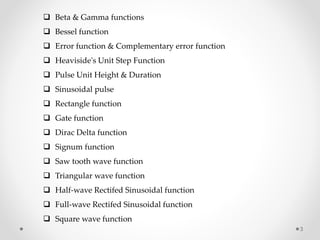Successfully reported this slideshow.

# Ic batch b1 sem 3(2015) introduction to some special functions and fourier series & fourier integral

2

Share×
1 of 30
1 of 30

# Ic batch b1 sem 3(2015) introduction to some special functions and fourier series & fourier integral

2

Share

Some special functions and series.

Some special functions and series.

## More Related Content

### Related Books

Free with a 14 day trial from Scribd

See all

### Related Audiobooks

Free with a 14 day trial from Scribd

See all

### Ic batch b1 sem 3(2015) introduction to some special functions and fourier series & fourier integral

1. 1. SHANTILAL SHAH GOVERNMENT ENGINEERING COLLEGE Postal Address : New Sidsar Campus Post : Vartej, Sidsar, Bhavnagar-364060, Gujarat, India Contact : 0278-2445509,2445767 Fax : 0278-2445509 Website: http://www.ssgec.ac.in/ Sr.no Roll no. Enrollment no. Name 1. 3017 140430117019 JITIN J PILLAI 2. 3010 140430117012 DODIYA PARTH 3. 3007 140430117008 CHAUHAN ASHISH 4. 3018 140430117020 JOSHI MEET 5. 3002 140430117002 BAVISHI BIRJU SEMESTER: 3RD BRANCH : INSTRUMENTATION & CONTROL BATCH : B1 Group members : Enrollment no.
2. 2. INTRODUCTION TO SOME SPECIAL FUNCTIONS 2
3. 3.  Beta & Gamma functions  Bessel function  Error function & Complementary error function  Heaviside's Unit Step Function  Pulse Unit Height & Duration  Sinusoidal pulse  Rectangle function  Gate function  Dirac Delta function  Signum function  Saw tooth wave function  Triangular wave function  Half-wave Rectifed Sinusoidal function  Full-wave Rectifed Sinusoidal function  Square wave function 3
4. 4. Beta function Bessel function Gamma functions 4
5. 5. Error function 5
6. 6. Complementary error function 6
7. 7. Heaviside's Unit Step Function 7
8. 8. Pulse Unit Height & Duration Sinusoidal Pulse 8
9. 9. Rectangle function Gate function 9
10. 10. Dirac Delta function 10
11. 11. Signum function 11
12. 12. Saw tooth wave function 12
13. 13. Triangular wave function Half-wave Rectifed Sinusoidal function 13
14. 14. Full-wave Rectifed Sinusoidal function Square wave function 14
15. 15. FOURIER SERIES & FOURIER INTEGRAL 15
16. 16. 16  Periodic function  Trigonometric series  Fourier series  Even and odd functions  Half-range Expansion  Applications of Fourier series: Forced oscillations  Fourier integral
17. 17. A function f is said to be periodic with period P (P being a nonzero constant) if we have for all values of x in the domain. Periodic function Trigonometric series A trigonometric series is a series of the form: It is called a Fourier series if the terms and have the form: where f is an integrable function. 17
18. 18. 18 A Fourier series is an expansion of a periodic function f(x) in terms of an infinite sum of sines and cosines. Fourier series With a Fourier series we are going to try to write a series representation for on in the form, So, a Fourier series is, in some way a combination of the Fourier sine and Fourier cosine series.
19. 19. 19 Even and Odd Functions They are special types of functions Even Functions A function is "even" when: f(x) = f(−x) for all x Odd Functions A function is "odd" when: −f(x) = f(−x) for all x
20. 20. 20 If a function is defined over half the range, say 0 to L, instead of the full range from −L to L, it may be expanded in a series of sine terms only or of cosine terms only. The series produced is then called a Half Range Fourier series. Half-range Expansion Conversely, the Fourier Series of an even or odd function can be analysed using the half range definition. An even function can be expanded using half its range from 0 to L or −L to 0 or L to 2L That is, the range of integration is L. Even function Half-range Expansion
21. 21. 21 The Fourier series of the half range even function is given by: for n=1,2,3,... , where and bn=0
22. 22. 22 Odd function Half-range Expansion An odd function can be expanded using half its range from 0 to L, i.e. the range of integration has value L. The Fourier series of the odd function is: Since ao = 0 and an = 0, we have:
23. 23. 23 Applications of Fourier series : Forced Oscillations  Consider a mass-spring system as before, where we have a mass m on a spring with spring constant k , with damping c , and a force F (t) applied to the mass. Suppose the forcing function F (t) is 2L -- periodic for some L > 0 . The equation that governs this particular setup is :
24. 24. 24  The general solution consists of the complementary solution xc , which solves the associated homogeneous equation mx ′′ + cx′ + kx = 0 , and a particular solution we call xp .  For c > 0 , the complementary solution xc will decay as time goes by. Therefore, we are mostly interested in a particular solution xp that does not decay and is periodic with the same period as F (t) .  We call this particular solution the steady periodic solution and we write it as xsp as before. What will be new in this section is that we consider an arbitrary forcing function F (t) instead of a simple cosine.  For simplicity, let us suppose that c = 0 . The problem with c > 0 is very similar. The equation has the general solution
25. 25. 25  In the spirit of the last section and the idea of undetermined coefficients we first write  Then we write a proposed steady periodic solution x as where an and bn are unknowns. We plug x into the differential equation and solve for an and bn in terms of cn and dn .
26. 26. 26 Fourier integral Let f(x) be a decaying (non-periodic ) function • f(x) is given by “linear combination” of cos(λx) & sin(λx) with a continuous interval of frequencies: all real numbers λ ≥ 0.
27. 27. 27 Fourier integral: Fourier integral formula: Convergence Theorem:
28. 28. 28 References INTERNET : https://en.wikipedia.org/wiki/List_of_mathematical_series https://en.wikipedia.org/wiki/Fourier_series https://en.wikipedia.org/wiki/Fourier_integral_operator people.math.gatech.edu/~xchen/teach/real.../Fourier_integral_intro.pdf math.mit.edu/cse/websections/cse41.pdf www.jirka.org/diffyqs/htmlver/diffyqsse29.html https://people.math.osu.edu/kwa.1/notes/512_2.7.pdf http://physics.bu.edu/~pankajm/PY501/fourier.pdf http://mathworld.wolfram.com/PeriodicFunction.html http://tutorial.math.lamar.edu/Classes/DE/FourierSeries.aspx http://www.fourier-series.com/
29. 29. 29 BOOKS : • Elementary Differential Equations (8th Edition), by W. E. Boyce and R. DiPrima, John Wiley (2005). • Advanced Engineering Mathematics (9th Edition), by E. Kreyszig, Wiley- India (2013). • Advanced Engineering Mathematics, by Ravish R. Singh and Mukul Bhatt, Mcgraw Hill Education (India) Pvt. Ltd. References
30. 30. 30